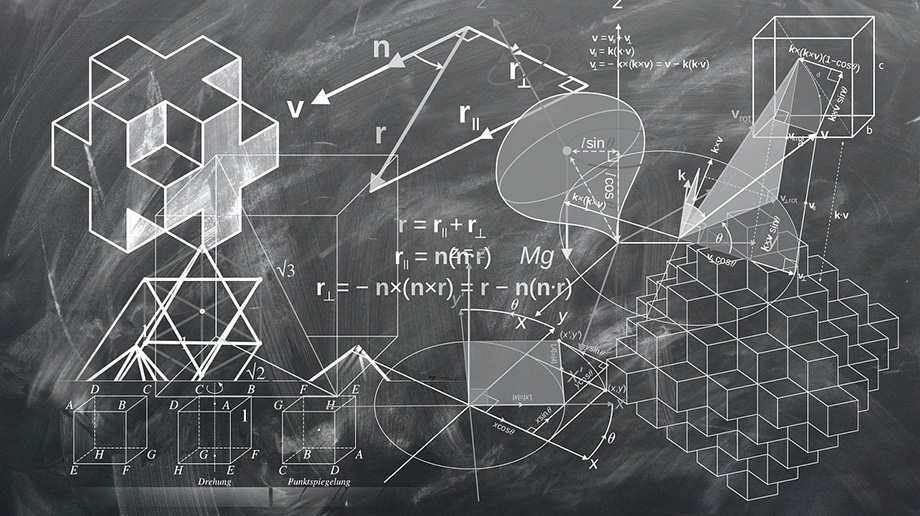# Volume Converter

Use this volume unit converter to easily convert the volume of an object, gas or liquid one measurement unit to another. Metric and imperial units are supported, including km3, m3, dm3, cm3, mm3, cubic miles, cubic yards, cubic feet, cubic inches, liters (litres), milliliters (ml), fluid gallons (US & Imperial), fluid quarts (US & Imperial), fluid pints (US & Imperial), fluid ounces (US & Imperial), as well as tablespoons and teaspoons, again, U.S. convention and Imperial convention.

Share converter:

Embed this tool:
get code

## What is Volume?

Volume is defined as the quantity which expresses the space occupied by a three-dimensional object, gas, or liquid. One can think of volume as the amount of water displaced by an objected fully submerged in a water tank. Understanding this is helpful in interepreting the output of our volume converter.

There are different ways to calculate the volumes of differently-shaped objects - you can check our volume calculators for formulas, references and easy calculation. Assuming you know or have measured the volume in one unit of volume, you might need to convert it to another, which is where our converter can be of assistance.

## Metric volume units

Volume units part of the International System of Measurements (SI) are based on the base unit: the meter, hence the name "metric system". Since every unit of length has a corresponding unit of volume (the corresponding volume of a cube with the given side length) the standard unit of volume in SI is the cubic meter (cubic metre in British English). Other units include the cubic kilometer, cubic decimeter, cubic centimeter, cubic millimeter, liter (UK: litre), milliliter, and others. Our volume converter supports all of these metrics. Below is a reference table for these units relative to the cubic meter.

Metric volume units (SI units)
UnitSymbolEquals
Cubic Kilometer km3 1,000,000,000 m3
Cubic Meter m3 1 m3
Liter / Litre l 1/1,000 m3
Cubic Decimeter dm3 1/100,000 m3
MilliLiter ml 1/1,000,000 m3
Cubic Centimeter cm3 1/10,000,000 m3
Cubic Millimeter mm3 1/1,000,000,000 m3

Cubic meters, centimeters, etc. are used to measure the volume of objects while liters and millileters are used for measuring the volume of gases and liquids. In the imperial system the cubic feet and more rarely the cubic yard are used for volumes of objects while liquid gallons, liquid quarts, pints, and fluid ounces are used for liquids and gases.Imperial units are more of a mess, customary, current and "legal" definitions conflicting with each other. Notably, the U.S. has a system separate from the Imperial one, used in the rest of the world, with the exception of the definition of tablespoons and teaspoons in Australia, which are on their own as well. You'd be happy to know our software supports most imperial units.

## Converting volume units

Converting between volume units is straightforward, especially if you have a calculator at hand, however, you need to know the conversion ratios for the different units. Below are several examples to help you get started but using our volume converter is by far the most convenient method.

## Volume conversion examples

### Cubic Meters to Cubic Feet

Sample task: convert 5 cubic meters to cubic feet. Solution:

Formula:
m3 * 35.315 = ft3
Calculation:
5 m3 * 35.3150 = 176.5750 ft3
End result:
5 m3 is equal to 176.5750 ft3

### Liters to US Liquid Gallons

Sample task: convert 100 liters (or litres) to gallons (US). Solution:

Formula:
l / 3.785 = g
Calculation:
100 l / 3.7850 = 26.420079 g
End result:
100 l is equal to 26.420079 g

### Fluid Ounces to Milliliters

Sample task: convert 10 fl oz (US) to milliliters (ml). Solution:

Formula:
fl oz * 29.574 = ml3
Calculation:
10 fl oz * 29.5740 = 295.74 ml3
End result:
10 fl oz is equal to 295.74 ml3

### Tablespoons (US) to Milliliters

Sample task: convert 2 tablespoons to ml. Solution:

Formula:
tbsp * 14.787 = ml
Calculation:
2 tbsp * 14.7870 = 29.5740 ml
End result:
2 tbsp is equal to 29.5740 ml